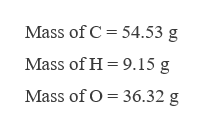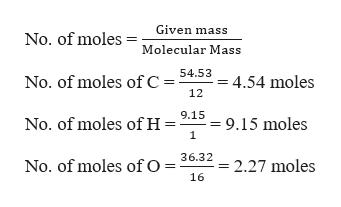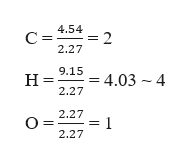# 1. Butyric acid is a fatty acid occurring in the form of esters in animal fats. A sample of butyric acid contains 54.53 g C, 9.15 g H, and 36.32 g O. The molar mass of butyric acid is 88.11 g/mol. Determine (a) and (b). Show your work. A. Empirical formula of butyric acid B. Molecular formula of butyric acid

Question
2 views

1. Butyric acid is a fatty acid occurring in the form of esters in animal fats. A sample of butyric acid contains 54.53 g C, 9.15 g H, and 36.32 g O. The molar mass of butyric acid is 88.11 g/mol. Determine (a) and (b). Show your work.

A. Empirical formula of butyric acid

B. Molecular formula of butyric acid

check_circle

Step 1

It is required to calculate the empirical and molecular formula of butyric acid.help_outlineImage TranscriptioncloseMass of C = 54.53 g Mass of H = 9.15 g Mass of O = 36.32 g fullscreen
Step 2

Calculating the number of moles of each element present,help_outlineImage TranscriptioncloseGiven mass No. of moles Molecular Mass 54.53 No. of moles of C 4.54 moles 12 9.15 := 9.15 moles No. of moles of H= 36.32 = 2.27 moles No. of moles of O = 16 fullscreen
Step 3

To obtain the empirical formula, divide all the values by the smallest...help_outlineImage Transcriptionclose4.54 =2 2.27 9.15 = 4.03 - 4 н 2.27 2.27 = 1 2.27 fullscreen

### Want to see the full answer?

See Solution

#### Want to see this answer and more?

Solutions are written by subject experts who are available 24/7. Questions are typically answered within 1 hour.*

See Solution
*Response times may vary by subject and question.
Tagged in

### General Chemistry